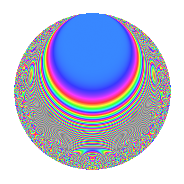# Properties

 Label 1006.2.aLevel 1006 Weight 2 Character orbit a Rep. character $$\chi_{1006}(1,\cdot)$$ Character field $$\Q$$ Dimension 41 Newforms 10 Sturm bound 252 Trace bound 3

# Related objects

## Defining parameters

 Level: $$N$$ = $$1006 = 2 \cdot 503$$ Weight: $$k$$ = $$2$$ Character orbit: $$[\chi]$$ = 1006.a (trivial) Character field: $$\Q$$ Newforms: $$10$$ Sturm bound: $$252$$ Trace bound: $$3$$ Distinguishing $$T_p$$: $$3$$, $$5$$

## Dimensions

The following table gives the dimensions of various subspaces of $$M_{2}(\Gamma_0(1006))$$.

Total New Old
Modular forms 128 41 87
Cusp forms 125 41 84
Eisenstein series 3 0 3

The following table gives the dimensions of the cuspidal new subspaces with specified eigenvalues for the Atkin-Lehner operators and the Fricke involution.

$$2$$$$503$$FrickeDim.
$$+$$$$+$$$$+$$$$8$$
$$+$$$$-$$$$-$$$$13$$
$$-$$$$+$$$$-$$$$12$$
$$-$$$$-$$$$+$$$$8$$
Plus space$$+$$$$16$$
Minus space$$-$$$$25$$

## Trace form

 $$41q$$ $$\mathstrut -\mathstrut q^{2}$$ $$\mathstrut -\mathstrut 4q^{3}$$ $$\mathstrut +\mathstrut 41q^{4}$$ $$\mathstrut -\mathstrut 4q^{7}$$ $$\mathstrut -\mathstrut q^{8}$$ $$\mathstrut +\mathstrut 41q^{9}$$ $$\mathstrut +\mathstrut O(q^{10})$$ $$41q$$ $$\mathstrut -\mathstrut q^{2}$$ $$\mathstrut -\mathstrut 4q^{3}$$ $$\mathstrut +\mathstrut 41q^{4}$$ $$\mathstrut -\mathstrut 4q^{7}$$ $$\mathstrut -\mathstrut q^{8}$$ $$\mathstrut +\mathstrut 41q^{9}$$ $$\mathstrut -\mathstrut 4q^{10}$$ $$\mathstrut +\mathstrut 4q^{11}$$ $$\mathstrut -\mathstrut 4q^{12}$$ $$\mathstrut -\mathstrut 6q^{13}$$ $$\mathstrut -\mathstrut 8q^{15}$$ $$\mathstrut +\mathstrut 41q^{16}$$ $$\mathstrut -\mathstrut 10q^{17}$$ $$\mathstrut -\mathstrut 5q^{18}$$ $$\mathstrut -\mathstrut 6q^{19}$$ $$\mathstrut -\mathstrut 8q^{21}$$ $$\mathstrut -\mathstrut 12q^{22}$$ $$\mathstrut -\mathstrut 20q^{23}$$ $$\mathstrut +\mathstrut 23q^{25}$$ $$\mathstrut -\mathstrut 2q^{26}$$ $$\mathstrut -\mathstrut 28q^{27}$$ $$\mathstrut -\mathstrut 4q^{28}$$ $$\mathstrut +\mathstrut 8q^{29}$$ $$\mathstrut -\mathstrut 4q^{30}$$ $$\mathstrut -\mathstrut 8q^{31}$$ $$\mathstrut -\mathstrut q^{32}$$ $$\mathstrut -\mathstrut 6q^{34}$$ $$\mathstrut -\mathstrut 4q^{35}$$ $$\mathstrut +\mathstrut 41q^{36}$$ $$\mathstrut -\mathstrut 16q^{37}$$ $$\mathstrut +\mathstrut 10q^{38}$$ $$\mathstrut -\mathstrut 8q^{39}$$ $$\mathstrut -\mathstrut 4q^{40}$$ $$\mathstrut +\mathstrut 2q^{41}$$ $$\mathstrut +\mathstrut 4q^{42}$$ $$\mathstrut +\mathstrut 4q^{43}$$ $$\mathstrut +\mathstrut 4q^{44}$$ $$\mathstrut +\mathstrut 4q^{46}$$ $$\mathstrut +\mathstrut 24q^{47}$$ $$\mathstrut -\mathstrut 4q^{48}$$ $$\mathstrut +\mathstrut 53q^{49}$$ $$\mathstrut -\mathstrut 7q^{50}$$ $$\mathstrut +\mathstrut 8q^{51}$$ $$\mathstrut -\mathstrut 6q^{52}$$ $$\mathstrut +\mathstrut 4q^{53}$$ $$\mathstrut +\mathstrut 12q^{54}$$ $$\mathstrut +\mathstrut 16q^{55}$$ $$\mathstrut +\mathstrut 20q^{57}$$ $$\mathstrut -\mathstrut 20q^{58}$$ $$\mathstrut +\mathstrut 12q^{59}$$ $$\mathstrut -\mathstrut 8q^{60}$$ $$\mathstrut -\mathstrut 2q^{61}$$ $$\mathstrut -\mathstrut 4q^{62}$$ $$\mathstrut +\mathstrut 8q^{63}$$ $$\mathstrut +\mathstrut 41q^{64}$$ $$\mathstrut +\mathstrut 44q^{65}$$ $$\mathstrut +\mathstrut 24q^{66}$$ $$\mathstrut -\mathstrut 24q^{67}$$ $$\mathstrut -\mathstrut 10q^{68}$$ $$\mathstrut +\mathstrut 40q^{69}$$ $$\mathstrut -\mathstrut 24q^{70}$$ $$\mathstrut +\mathstrut 12q^{71}$$ $$\mathstrut -\mathstrut 5q^{72}$$ $$\mathstrut -\mathstrut 14q^{73}$$ $$\mathstrut +\mathstrut 16q^{74}$$ $$\mathstrut -\mathstrut 56q^{75}$$ $$\mathstrut -\mathstrut 6q^{76}$$ $$\mathstrut +\mathstrut 4q^{77}$$ $$\mathstrut +\mathstrut 4q^{78}$$ $$\mathstrut +\mathstrut 4q^{79}$$ $$\mathstrut +\mathstrut 9q^{81}$$ $$\mathstrut -\mathstrut 30q^{82}$$ $$\mathstrut +\mathstrut 40q^{83}$$ $$\mathstrut -\mathstrut 8q^{84}$$ $$\mathstrut -\mathstrut 16q^{85}$$ $$\mathstrut -\mathstrut 20q^{86}$$ $$\mathstrut -\mathstrut 8q^{87}$$ $$\mathstrut -\mathstrut 12q^{88}$$ $$\mathstrut +\mathstrut 2q^{89}$$ $$\mathstrut +\mathstrut 8q^{90}$$ $$\mathstrut -\mathstrut 28q^{91}$$ $$\mathstrut -\mathstrut 20q^{92}$$ $$\mathstrut +\mathstrut 24q^{93}$$ $$\mathstrut +\mathstrut 4q^{94}$$ $$\mathstrut -\mathstrut 36q^{95}$$ $$\mathstrut -\mathstrut 66q^{97}$$ $$\mathstrut +\mathstrut 7q^{98}$$ $$\mathstrut +\mathstrut 60q^{99}$$ $$\mathstrut +\mathstrut O(q^{100})$$

## Decomposition of $$S_{2}^{\mathrm{new}}(\Gamma_0(1006))$$ into irreducible Hecke orbits

Label Dim. $$A$$ Field CM Traces A-L signs $q$-expansion
$$a_2$$ $$a_3$$ $$a_5$$ $$a_7$$ 2 503
1006.2.a.a $$1$$ $$8.033$$ $$\Q$$ None $$-1$$ $$0$$ $$0$$ $$0$$ $$+$$ $$-$$ $$q-q^{2}+q^{4}-q^{8}-3q^{9}+4q^{11}+2q^{13}+\cdots$$
1006.2.a.b $$1$$ $$8.033$$ $$\Q$$ None $$-1$$ $$1$$ $$-2$$ $$1$$ $$+$$ $$+$$ $$q-q^{2}+q^{3}+q^{4}-2q^{5}-q^{6}+q^{7}+\cdots$$
1006.2.a.c $$1$$ $$8.033$$ $$\Q$$ None $$1$$ $$-3$$ $$0$$ $$-3$$ $$-$$ $$-$$ $$q+q^{2}-3q^{3}+q^{4}-3q^{6}-3q^{7}+q^{8}+\cdots$$
1006.2.a.d $$1$$ $$8.033$$ $$\Q$$ None $$1$$ $$-1$$ $$0$$ $$1$$ $$-$$ $$-$$ $$q+q^{2}-q^{3}+q^{4}-q^{6}+q^{7}+q^{8}+\cdots$$
1006.2.a.e $$1$$ $$8.033$$ $$\Q$$ None $$1$$ $$1$$ $$-4$$ $$1$$ $$-$$ $$-$$ $$q+q^{2}+q^{3}+q^{4}-4q^{5}+q^{6}+q^{7}+\cdots$$
1006.2.a.f $$2$$ $$8.033$$ $$\Q(\sqrt{5})$$ None $$-2$$ $$0$$ $$-2$$ $$-4$$ $$+$$ $$+$$ $$q-q^{2}-\beta q^{3}+q^{4}+(-1-\beta )q^{5}+\beta q^{6}+\cdots$$
1006.2.a.g $$5$$ $$8.033$$ 5.5.36497.1 None $$-5$$ $$0$$ $$-1$$ $$3$$ $$+$$ $$+$$ $$q-q^{2}+(\beta _{1}-\beta _{3}+\beta _{4})q^{3}+q^{4}+(\beta _{2}+\cdots)q^{5}+\cdots$$
1006.2.a.h $$5$$ $$8.033$$ 5.5.205225.1 None $$5$$ $$-4$$ $$-3$$ $$-9$$ $$-$$ $$-$$ $$q+q^{2}+(-1+\beta _{1})q^{3}+q^{4}+(-1-\beta _{1}+\cdots)q^{5}+\cdots$$
1006.2.a.i $$12$$ $$8.033$$ $$\mathbb{Q}[x]/(x^{12} - \cdots)$$ None $$-12$$ $$-3$$ $$7$$ $$-2$$ $$+$$ $$-$$ $$q-q^{2}-\beta _{4}q^{3}+q^{4}+(\beta _{7}-\beta _{10})q^{5}+\cdots$$
1006.2.a.j $$12$$ $$8.033$$ $$\mathbb{Q}[x]/(x^{12} - \cdots)$$ None $$12$$ $$5$$ $$5$$ $$8$$ $$-$$ $$+$$ $$q+q^{2}+\beta _{1}q^{3}+q^{4}-\beta _{9}q^{5}+\beta _{1}q^{6}+\cdots$$

## Decomposition of $$S_{2}^{\mathrm{old}}(\Gamma_0(1006))$$ into lower level spaces

$$S_{2}^{\mathrm{old}}(\Gamma_0(1006)) \cong$$ $$S_{2}^{\mathrm{new}}(\Gamma_0(503))$$$$^{\oplus 2}$$